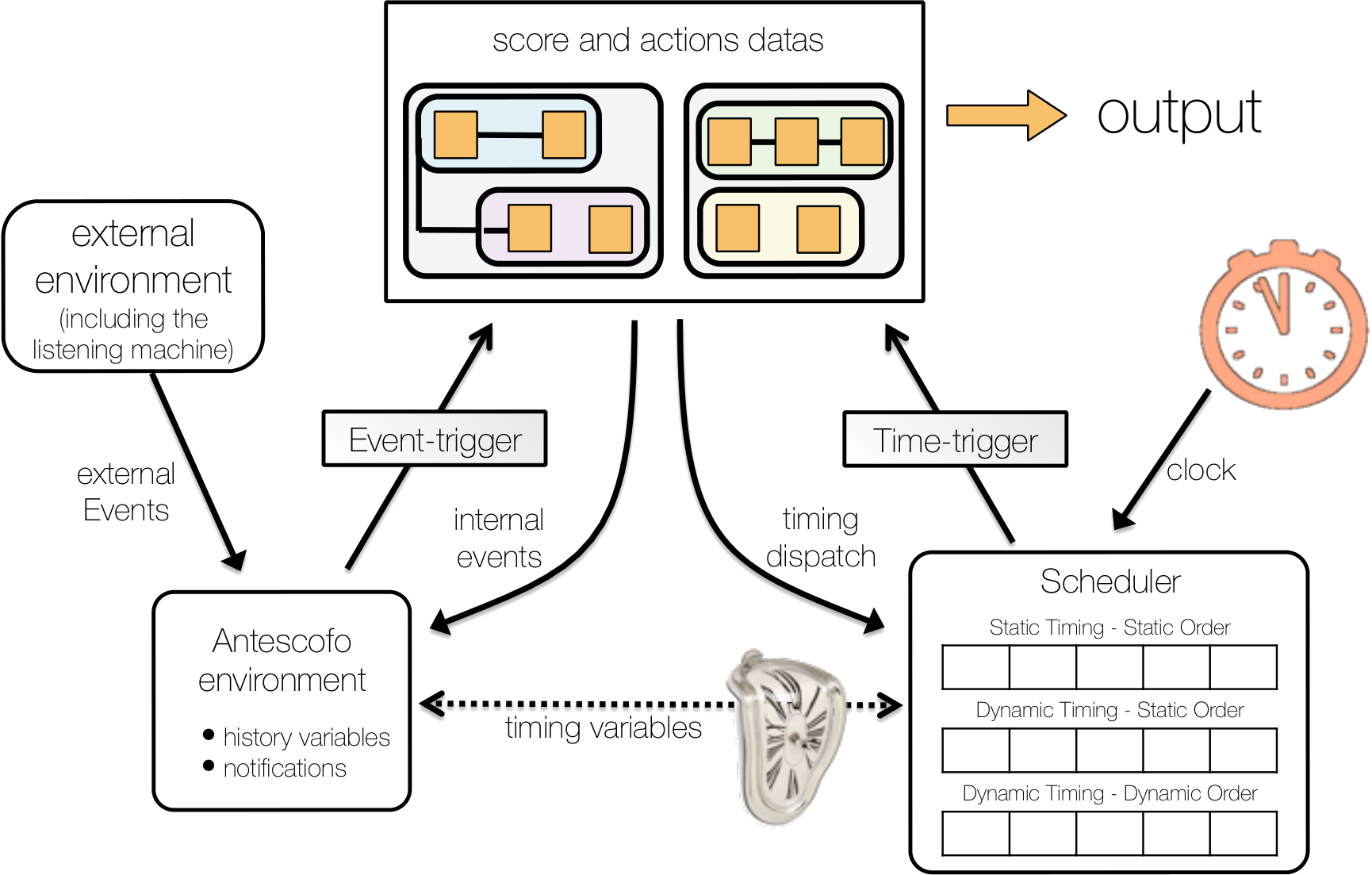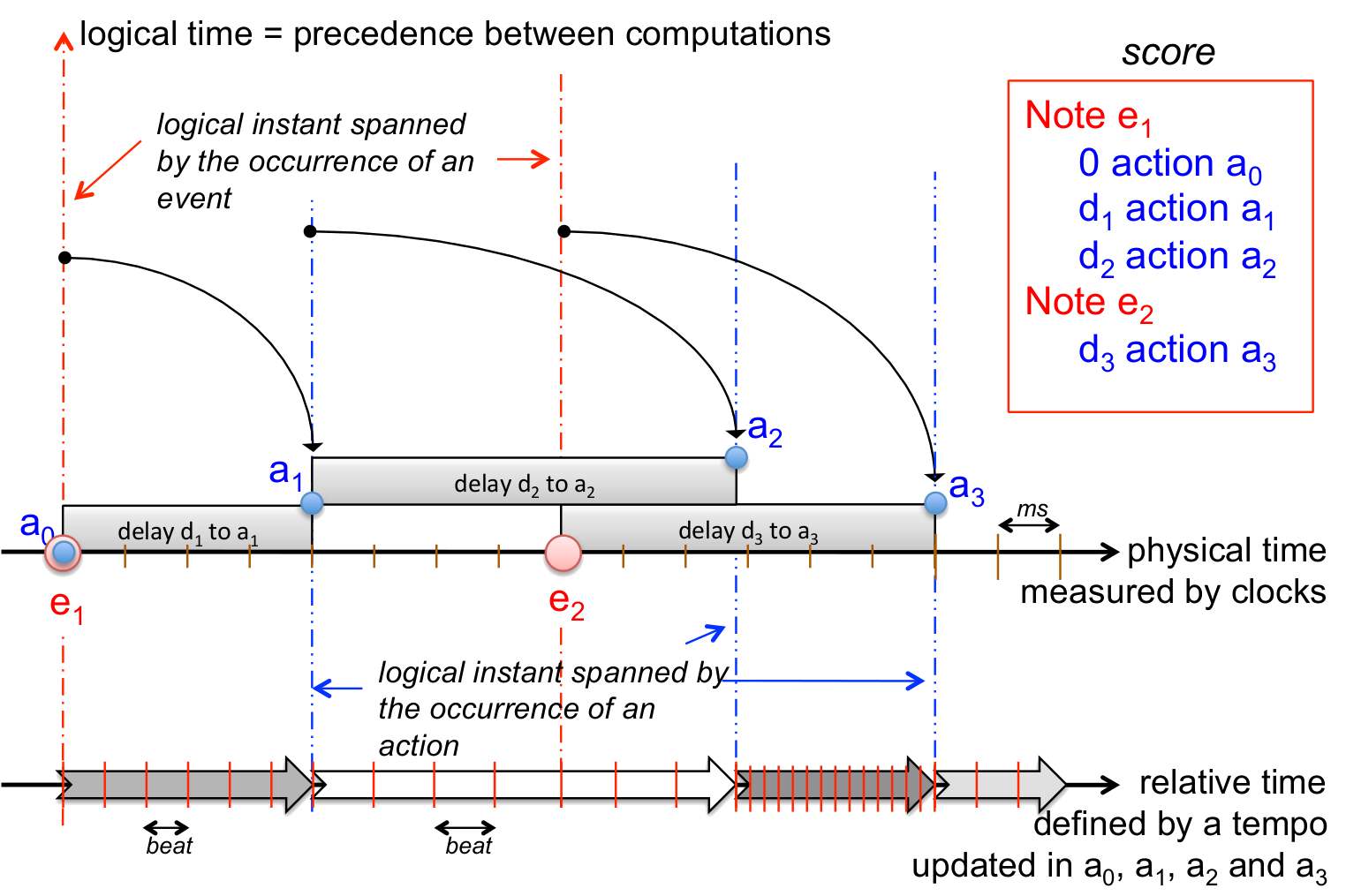# Management of Time¶

The language developed in Antescofo can be seen as a domain specific synchronous and timed reactive language in which the accompaniment actions of a mixed score are specified together with the instrumental part to follow. It thus takes care of timely delivery, coordination and synchronisation of actions with regards to the external environment (musicians) using machine listening.

Experienced users should note that is delivered with its own Real-time Scheduler. This is mainly to reduce utility costs of using internal Max and Pd timers and to significantly reduce their interference with other actions in Max/Pd schedulers themselves. The internal scheduler is new since version $0.5$ onwards. It is sketched briefly in this chapter.

Actions are computations triggered after a delay that elapses starting from the occurrence of an event or another action. In this way, Antescofo is both a reactive system, where computations are triggered by the occurrence of an event, and a timed system, where computations are triggered at some date. The three main components of the system architecture are sketched in the figure below:

• The scheduler takes care of the various time coordinate specified in the score and manage all delays, wait time and pending tasks.

• The environment handle the memory store of the system: the history of the variables, the management of references and all the notification and event signalization.

• The evaluation engine is in charge of parsing the score and of the instantaneous evaluation of the expressions and of the actions.An action is launched because the recognition of a musical event, a notification of the external environment (i.e., external assignment of a variable or the reception of an OSC message), internal variable assignment by the functioning of the program itself, or the expiration of a delay. Actions are launched after a delay which can be expressed in various time coordinate.

There are several temporal coordinate systems that can be used to locate the occurrence of an event or an action and to define a duration.

## Logical Instant¶

A logical instant is an instant in time distinguished because it corresponds to:

• the recognition of a musical event;

• the assignment of a variable by the external environment (e.g. through an OSC message or a MAX/PD binding);

• the expiration of a delay.

Such an instant has a date (i.e. a coordinate) in each time coordinate system. The notion of logical instants is instrumental to maintaining the synchronous abstraction of actions (i.e. the idea that two actions may occur simultaneously) and to reduce temporal approximation. Whenever a logical instant is started, the internal variables $NOW (current date in the physical time frame) and (current date in the relative time frame) $RNOW are updated, see section system variables. Within the same logical instant, synchronous actions are performed sequentially in the same order as in the score.

Computations are supposed to take no time and thus, atomic actions are performed inside one logical instant of zero duration. This abstraction is a useful simplification to understand the scheduling of actions in a score. In the real world, computations take time but this time can be usually ignored and does not disturb the scheduling planned at the score level.

In the figure below, the sequence of synchronous actions appears in the vertical axis. So this axis corresponds to the dependency between simultaneous computations. Notice that the (vertical) height of a box is used to represent the logical dependencies while the (horizontal) length of a box represents a duration in time. Note for example that even if durations of $a_1$ and $a_2$ are both zero, the execution order of actions $a_0$, $a_1$ and $a_2$ is the same as the appearance order in the score.## Time Coordinate¶

A time coordinate system is used to interpret delays and to give a date to the occurrence of an event or to the launching of an action. Two frames of reference are commonly used:

• the physical time $\mathcal{P}$ expressed in seconds and measured by a clock (also called wall clock time),

• and the relative time which measure the progression of the performance in the score or in a sequence of actions. The position in the score is measured in beats. The relative time is relative to a tempo.

A tempo specifies the “passing of time” relatively to the physical time1. In short, a tempo is expressed as a number of beats per minutes.

Programmers may introduce their own tempo local to a group of actions using a dedicated attribute @tempo. The corresponding coordinate systems is then used for all relative delays and datation used in the actions within this group. The tempo expression is evaluated continuously in time for dynamically computing the relationships specified by equation $$t_\mathcal{T} = \int_0^{t_\mathcal{P}} T_\mathcal{T}$$
linking the date $t_\mathcal{P}$ of the occurrence of an event in the physical time and the date $t_\mathcal{T}$ of the same event in the relative time $\mathcal{T}$.

Antescofo provides a predefined dynamic tempo variable through the system variable $RT_TEMPO. This tempo is refered as “the tempo” and has a tremendous importance because it is the time frame naturally associated with the musician part of the score2. This variable is extracted from the audio stream by the listening machine, relying on cognitive model of musician behavior3. The corresponding time coordinate system is used when we speak of “relative time” without additional qualifier. ## Locating an Action in Time¶ Given a time coordinate, there are several ways to implement the specification of the occurrence of an action. For instance, consider action $a_2$ in the above figure and suppose that $d_1 + d_2$ is greater than $1.5$ beat (the duration of the event NOTE e₁. Then action $a_2$ can be launched either: • $(d_1 + d_2)$ beats after the occurrence of the event NOTE e₁; • or $(d_1 + d_2 - 1.5)$ beats after the occurrence of the event NOTE e₂. (several other variations are possible). In the “ideal interpretation of the score”, these two ways of computing the launching date of action $a_2$ are equivalent because the event NOTE e₂ occurs exactly after $1.5$ beat after event NOTE e₁. But this is not the case in an actual performance. Antescofo allows a composer to choose the right way to compute the date of an action in a time frame, to best match the musical context. This is the purpose of the Synchronization Strategies. 1. Mazzola, G., & Zahorka, O. (1994). Tempo curves revisited: Hierarchies of performance fields. Computer Music Journal, 18(1), 40-52. 2. The infered tempo is computed by Antescofo to mimics the tracking of the tempo by a human, and implements an idea of smooth tempo fluctuation, rather than trying to satisfy exactly the above equation at any moment. So, for the relative time frame, the previous equation is only an approximation. As a consequence, the current position in the score is explicitly given by the variable $BEAT_POS which is more accurate than the integration of \$RT_TEMPO

3. A. Cont. A coupled duration-focused architecture for realtime music to score alignment. IEEE Transaction on Pattern Analysis and Machine Intelligence, Juin 2010, Vol. 32, n$\circ$6, pp 974–987.